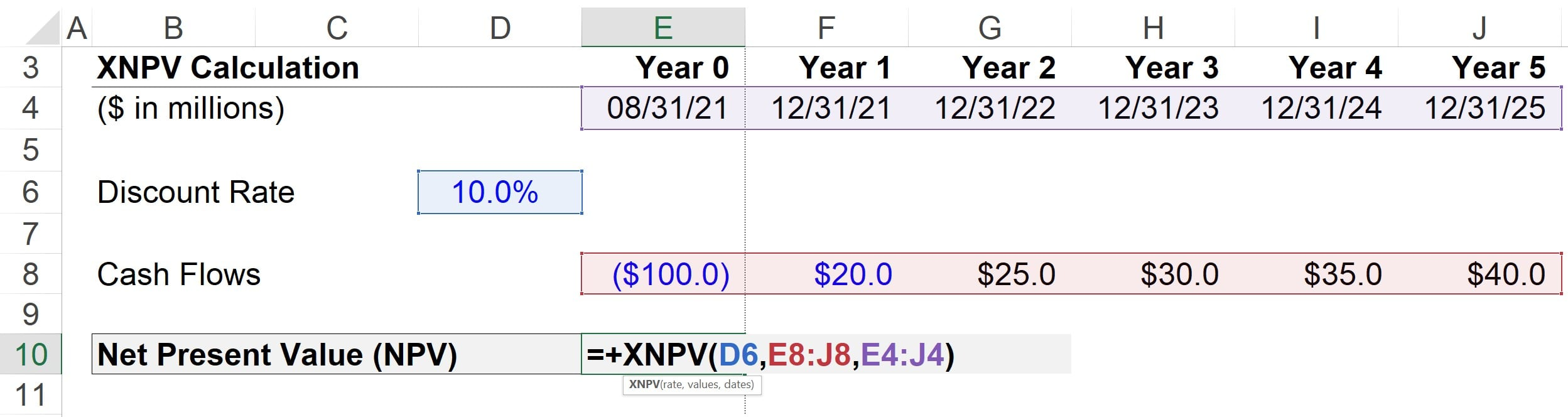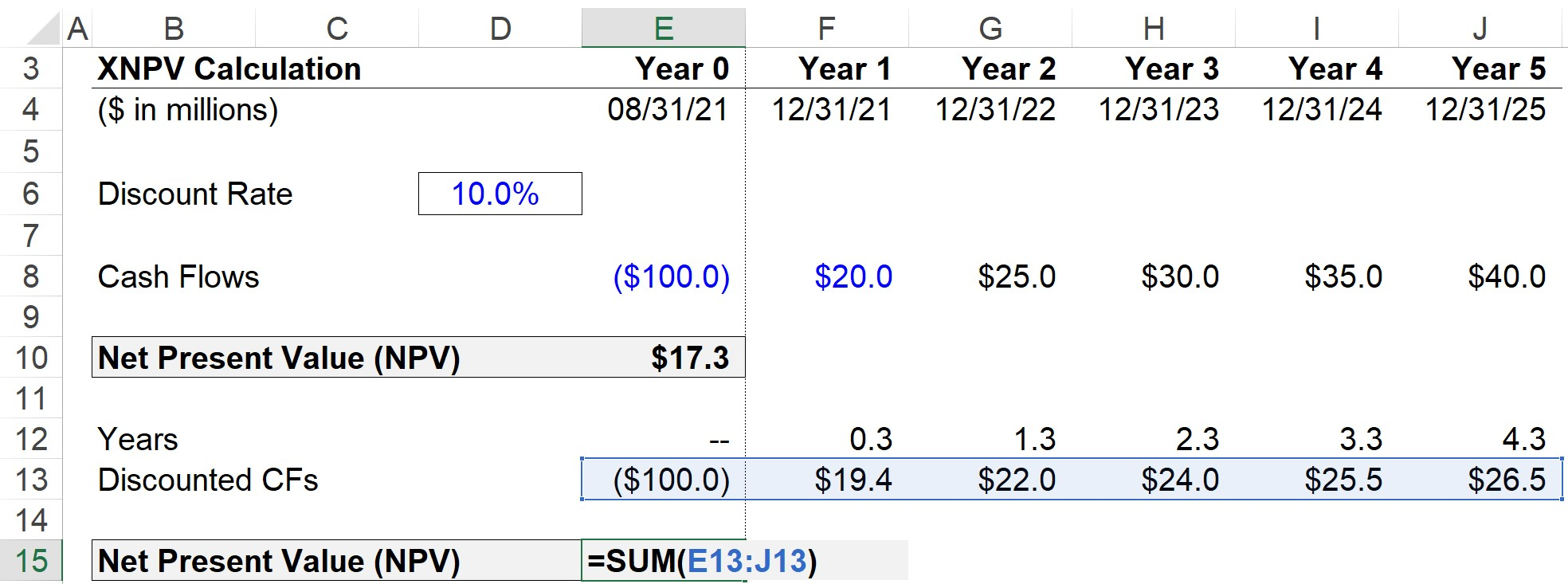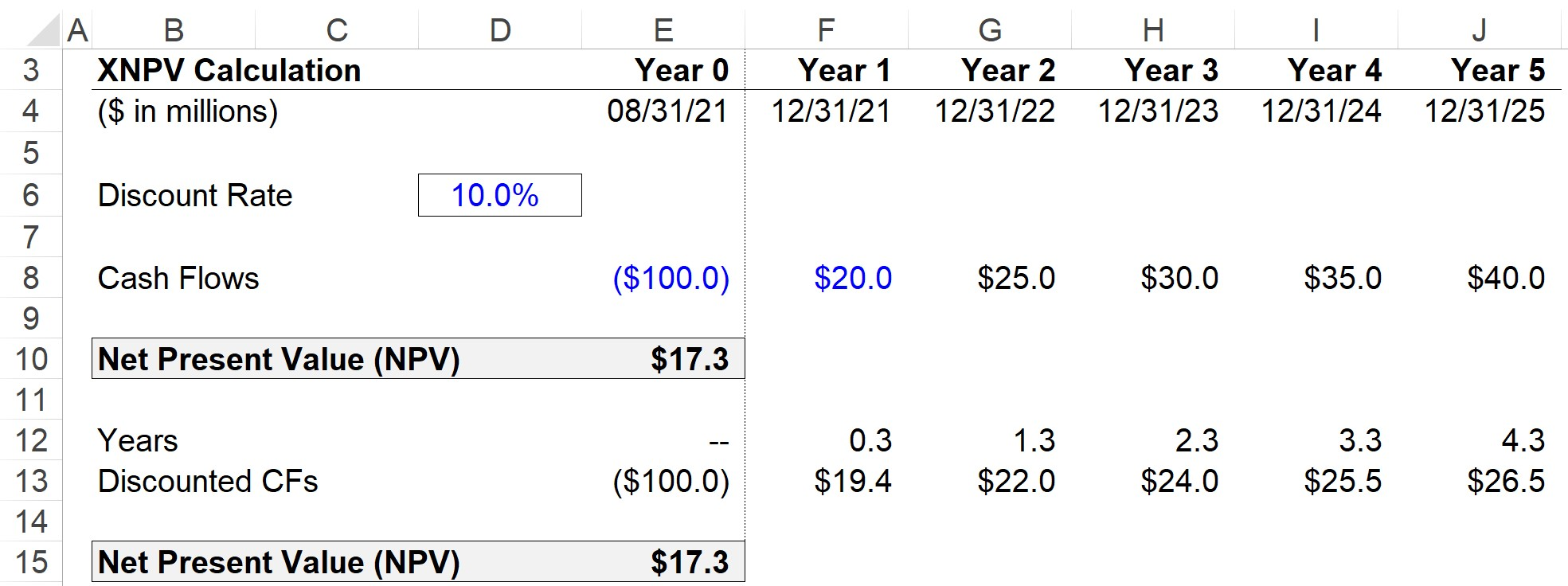Welcome to Wall Street Prep! Use code at checkout for 15% off.# Net Present Value (NPV)

Guide to Understanding Net Present Value (NPV)## How to Calculate NPV?

The net present value (NPV) represents the discounted values of future cash inflows and outflows related to a specific investment or project.

The present value (PV) of a stream of cash flows refers to the value of the future cash flows as of the current date.

Since a dollar received today is worth more than a dollar received on a later date because of the “time value of money”, future cash flows must be discounted to the present date using an appropriate rate of return, i.e. the discount rate.

Performing NPV analysis is a practical method to determine the economic feasibility of undertaking a potential project or investment.

The discounted cash flows are inclusive of the cash inflows and cash outflows; hence, the usefulness of the metric in capital budgeting.

To calculate the net present value (NPV), our recommendation is to use the XNPV function in Excel.

Unlike the NPV function in Excel – which assumes the time periods are equal – the XNPV function takes into account the specific dates that correspond to each cash flow.

Therefore, XNPV is a more practical measure of NPV, considering cash flows are usually generated at irregular intervals.

## NPV Formula

The Excel formula for XNPV is as follows:

=XNPV(Rate, Values, Dates)

Where:

• Rate → The appropriate discount rate based on the riskiness and potential returns of the cash flows
• Values → The array of cash flows, with all cash outflows and inflows accounted for
• Dates → The corresponding dates for the series of cash flows that were selected in the “values” array

## What is a Good NPV? (Positive vs. Negative)

On the topic of capital budgeting, the general rules of thumb to follow for interpreting the net present value (NPV) of a project or investment is as follows.

• If NPV > 0: Accept (Profitable)
• If NPV = 0: Indifferent (Break-Even Point)
• If NPV < 0: Reject (Unprofitable)

If the net present value is positive, the likelihood of accepting the project is far greater.

Why?

A project or investment with a positive NPV is implied to create positive economic value, whereas one with a negative NPV is anticipated to destroy value.

But please note the following guidelines mentioned earlier are generalizations and are not meant to be rigid rules.

For example, a project could be unprofitable yet still be accepted by management if there are other non-monetary considerations (e.g. intangible factors such as marketing/publicity, and relationship-building) that help rationalize the decision.

## NPV Calculator – Excel Template

We’ll now move to a modeling exercise, which you can access by filling out the form below.Submitting...

## 1. Capital Budgeting Project Assumptions

Suppose a corporation is attempting to decide whether to accept or decline a proposed project.

The initial investment of the project in Year 0 amounts to \$100m, while the cash flows generated by the project will begin at \$20m in Year 1 and increase by \$5m each year until Year 5.

The discount rate, date, and cash flow assumptions for calculating the net present value are listed below:

• Discount Rate = 10%
• Year 0 (8/31/21) = -\$100m
• Year 1 (12/31/21) = \$20m
• Year 2 (12/31/22) = \$25m
• Year 3 (12/31/23) = \$30m
• Year 4 (12/31/24) = \$35m
• Year 5 (12/31/25) = \$40m

The period from Year 0 to Year 1 is where the timing irregularity occurs (and why the XNPV is recommended over the NPV function).

## 2. NPV Analysis in Excel (XNPV Function)

Since we have all the necessary inputs, we can enter them into the formula presented earlier.Upon doing so, we get \$17.3m as the net present value (NPV).

## 3. NPV Calculation Example

Alternatively, we can also manually discount each of the cash flows by dividing the cash flow by (1 + discount rate) ^ the number of periods.

• Year 0: -\$100m ÷ (1+10%)^0.0 = -\$100.0m
• Year 1: \$20m ÷ (1+10%)^0.3 = \$19.4m
• Year 2: \$25m ÷ (1+10%)^1.3 = \$22.0m
• Year 3: \$30m ÷ (1+10%)^2.3 = \$24.0m
• Year 4: \$35m ÷ (1+10%)^3.3 = \$25.5m
• Year 5: \$40m ÷ (1+10%)^4.3 = \$26.5m

In Excel, the number of periods can be calculated using the “YEARFRAC” function and selecting the two dates (i.e. beginning and ending dates).If we calculate the sum of all cash inflows and outflows, we get \$17.3m once again for our NPV.

In closing, the project in our example exercise is more likely to be accepted because of its positive net present value (NPV).

• Accept or Reject Project? → “Accept”Step-by-Step Online Course

### Everything You Need To Master Financial Modeling

Enroll in The Premium Package: Learn Financial Statement Modeling, DCF, M&A, LBO and Comps. The same training program used at top investment banks.

Inline Feedbacks
Jaspreet singh
January 4, 2023 10:22 am